New update is available. Click here to update.

# Introduction of Time Complexity

## Introduction

This article aims to introduce you to the time complexity and the big O notations.

We will understand the time complexity and learn how to calculate the time complexity of an algorithm.

To brush up on your knowledge of the basic complexity, you can read the article Time and Space complexity on Codestudio.

## What is time complexity?

The amount of time an algorithm takes to run based on the input size is known as time complexity.

## Why do we need to find out the time complexity of an algorithm?

In computer science, a problem can be solved using different algorithms. Some algorithms may be high-speed, and some may be very slow. We must find out the fastest and most efficient algorithm to save time to solve the problem. To find out the fastest algorithm, we must understand the time complexity.

## The Big O notation

The time complexity is represented using the big O notation.

In the below table, you can see the big O notation and their meaning.

The time complexity and the Big O notation of some popular algorithms are given in the below table:

Also see, Morris Traversal for Inorder.

Also see, Longest Common Substring

## Let us understand the time complexity using examples

Consider a problem where we have to find if an element is present in an array sorted in ascending order. Consider N = length of the array.

We can find the element using linear search. In this method, we iterate through the array, and if we find the element, we stop and print the index. Using this method, we may have to iterate through the whole array, and so the time complexity is O(N).

We can also find the presence of the element using binary search. We go to the mid element, and if the target element is greater than the mid element, we search the target element in the right part of the array. Similarly, if the target element is less than the mid element, then we search the target element again in the left part of the array. Using this method, we can find if the target element is present in the array or not in O(log2(N)) time.

Here is the code for the same implementation:

``````def binary_search(arr,target):
low=0
high=len(arr)
while low<high:
mid=low+(high-low)//2
if arr[mid]==target:
return True
if arr[mid]>target:
high=mid
else:
low=mid+1
return False
present=binary_search([15,23,33,100,200,218,343,475, 555,700],343)
print(present) # True``````

Consider binary search in the below example:

arr=[15,23,33,100,200,218,343,475, 555,700]

Search_element=343

First, we go to the mid element as shown in the figure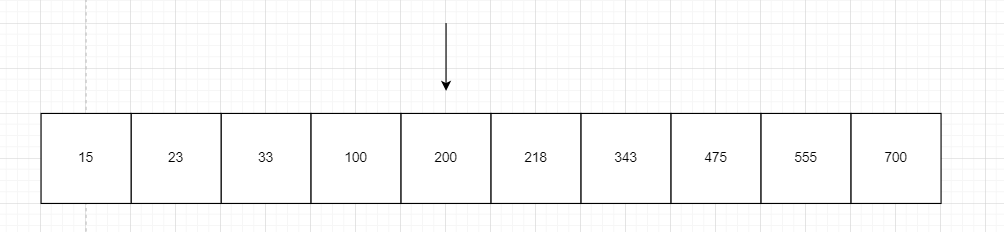Mid element: i.e. 200 in the array

Now as 343>200, it must be present on the right side of the 200.

So we again search for the mid element in the right side of the array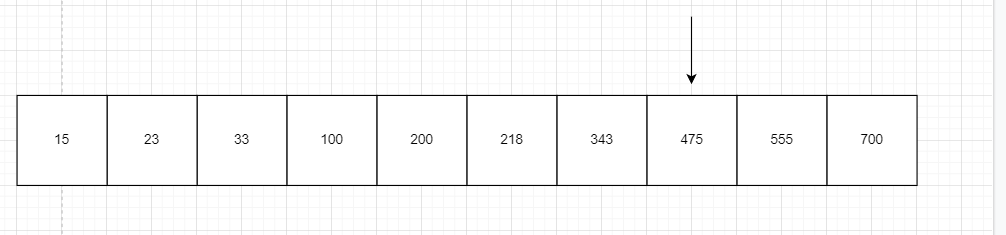Searching mid element on the right side of the array: 475

Now as mid_element=475>343, we search on the left side of 475 and right side of 200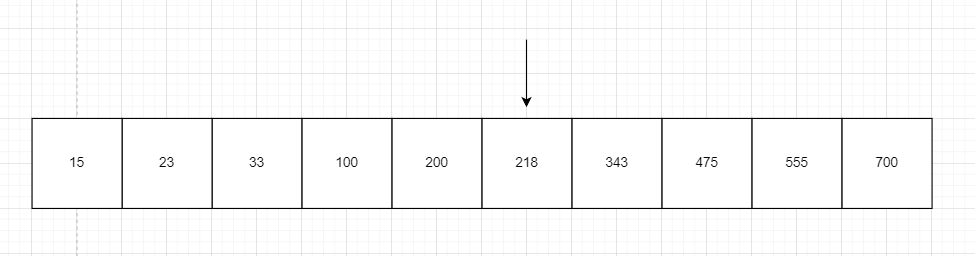Searching element 343 on the right of 200 and left of 475

Again 343> 218 so we search on the right side of 218 and left of 475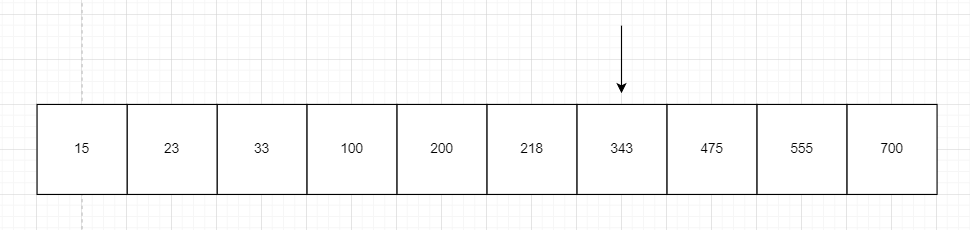Searching the element 343 on the right of 218 and left of 475

Now, 343=mid_element, thus we return True.

We can see that the array gets divided into two parts in each case. Thus the time complexity is O(log2(N)).

Thus we can find the search_element in O(N) time using linear search algorithm as well as in O(log(N)) using a binary search algorithm. The binary search algorithm works very fast on large arrays. Suppose an array has trillion elements, then the binary search takes only log2(N) = 40 operations which take less than a microsecond to calculate. In comparison, the linear search takes trillions of operations which is 1000s(considering one billion operations per second). Thus choosing an efficient algorithm saves us the most time.

## Here are some of the codes describing time complexity

Constant time complexity: Whenever a solution takes constant time to solve the problem, the time complexity is said to be O(1) or constant time complexity. Eg: the inbuilt max(a,b) function in c++ takes constant time to find out the maximum element among the two input elements.

``````#include<bits/stdc++.h>
using namespace std;
int main(){
int a=3,b=4;
cout<<max(a,b)<<endl; //output = 4
}``````

Logarithmic time complexity: If a solution takes O(log(N)) time complexity to solve the problem, the time complexity is said to be O(log(N)) or logarithmic time complexity. E.g., the binary_search function in c++ STL takes logarithmic time complexity to find out if a target element is present in the vector or not

``````#include<bits/stdc++.h>
using namespace std;
int main(){
vector<int> v={1,2,34,45,56};
cout<<binary_search(v.begin(),v.end(),2)<<endl; //output = 1 as 2 is in v
cout<<binary_search(v.begin(),v.end(),22)<<endl; //output = 0 as 22 is not in v
}``````

Linear time complexity: If a solution takes time in the multiple of N to solve the problem, the time complexity is said to be O(N) or linear time complexity. Eg: the algorithm that finds the sum of an array by iterating and adding all the elements has linear time complexity.

Before we proceed, let's watch the video below for a quick recap of how time complexity is calculated using live examples.

## Comparison of time complexity from best to worst

O(1) > O(log(n)) > O(n) > O(n x log(n)) O(n2) > O(n3) > O(2n) > O(n!) > O(nn)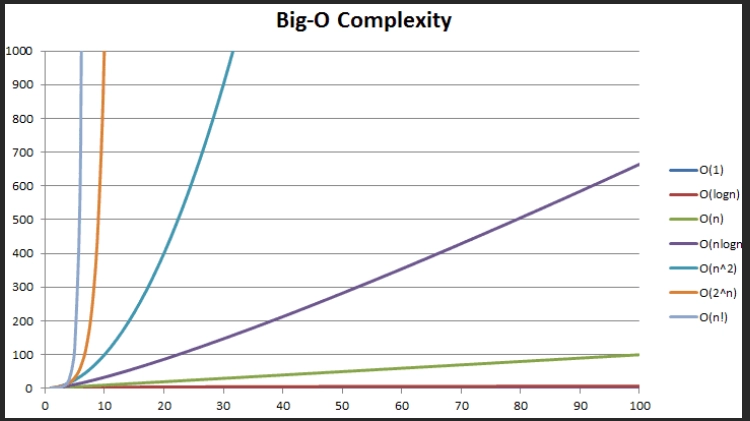Fig shows a comparison between different time complexity.

The time complexity of an algorithm that grows slowly with the increase in input size is considered better. For example, the logarithm function grows much more slowly than linear, so logarithmic time complexity is much better than linear time complexity.

## FAQs

1. What are some famous algorithms for finding the time complexity for a program?
We can use the master theorem to find the time complexity when a recurrence relation is given. There are other methods like substitution method, iteration method etc.

2. Which algorithm is better, one having time complexity O(2n) or one having time complexity O(n!)?
O(n!) grows much faster than O(2n). Also, we can see this from the graph given. Thus an algorithm with O(2n) time complexity is much better than an algorithm with factorial time complexity.

3. Which algorithm is better, one having time complexity O(n) or one having time complexity O(log(n)2)?
O(n) grows much faster than O(log(n)2). At n=million, the first one will take a million operations, whereas the latter will take 202 = 400 operations. Thus an algorithm having O(log(n)2) is much better than an algorithm having time complexity O(n)

4. What is the need to optimise time complexity?
A good program is always optimised and efficient.

5. How can we optimize time complexity in a program?
We can use proper data structure along with the appropriate algorithms to optimize the time complexity.

## Key Takeaways

We understand the basics of time complexity for codes and their importance for solving an algorithm. We saw different programs having different time complexity.

You can also continue reading further articles Time and space complexity and Trick Questions from time and space complexity on Codestudio.

Check out CodeStudio to learn more about competitive programming, DSA, attempt mock tests, interview preparation and much more.

Happy Learning!!!